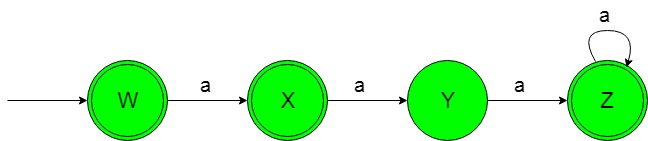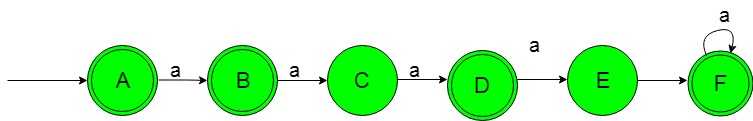# Designing Deterministic Finite Automata (Set 10)

Prerequisite: Designing finite automata
In this article, we will see some designing of Deterministic Finite Automata (DFA).

Problem-1: Construction of a minimal DFA accepting set of strings over {a} in which {an | n≥0, n≠2 i.e, ‘n’ should be greater than 0 and not equal to 2}.
Explanation: The desired language will be like:

`L1 = {ε, a, aaa, aaaa, aaaaa, ..................}`

Here ε is taken as string because value of ‘n’ is greater than or equal to zero and rest of the strings are having ‘a’ to the power of any positive natural number but not 2.
Below language is not accepted by this DFA because some of the string containing ‘a’ to the power 2.

`L2 = {aa, aaaaa, ..........}`

This language L2 is not accepted by this required DFA because of its string containing ‘a’ to the power of 2.
The state transition diagram of the desired language will be like below:In the above DFA, the initial and final state’W’ on getting ‘a’ as the input it transits to a final state ‘X’. The final state ‘X’ on getting ‘a’ as the input it transits to a state ‘Y’. The state ‘Y’ on getting ‘a’ as the input it transits to a final state ‘Z’ which on getting any number of ‘a’ it remains in the state of itself.

### Python Implementation:

 `             `  `def` `stateW(n): ` `    ``#if length of n become 0  ` `    ``#then print accepted ` `    ``if``(``len``(n)``=``=``0``): ` `        ``print``(``"string accepted"``) ` `         `  `    ``else``:  ` `        ``#if at zero index  ` `        ``#'a' found call ` `        ``#stateX function     ` `        ``if` `(n[``0``]``=``=``'a'``): ` `            ``stateX(n[``1``:]) ` `        `  `             `  `         `  `def` `stateX(n): ` `    ``#if length of n become 0  ` `    ``#then print accepted ` `    ``if``(``len``(n)``=``=``0``): ` `        ``print``(``"string accepted"``) ` `         `  `    ``else``:   ` `        ``#if at zero index  ` `        ``#'a' found call ` `        ``#stateY function     ` `        ``if` `(n[``0``]``=``=``'a'``): ` `            ``stateY(n[``1``:]) ` `         `  `def` `stateY(n): ` `    ``#if length of n become 0  ` `    ``#then print not accepted ` `    ``if``(``len``(n)``=``=``0``): ` `        ``print``(``"string not accepted"``) ` `         `  `    ``else``: ` `        ``#if at zero index  ` `        ``#'a' found call ` `        ``#stateZ function     ` `        ``if` `(n[``0``]``=``=``'a'``): ` `            ``stateZ(n[``1``:]) ` `         `  `def` `stateZ(n): ` `    ``#if length of n become 0  ` `    ``#then print accepted ` `    ``if``(``len``(n)``=``=``0``): ` `        ``print``(``"string accepted"``) ` `         `  `    ``else``:  ` `        ``#if at zero index  ` `        ``#'a' found call ` `        ``#stateZ function     ` `        ``if` `(n[``0``]``=``=``'a'``): ` `            ``stateZ(n[``1``:]) ` `             `  `#take input ` `n``=``input``() ` ` `  `#call stateW function ` `#to check the input ` `stateW(n) `

Problem-2: Construction of a minimal DFA accepting set of strings over {a} in which {an | n≥0, n≠3, n≠4 i.e, ‘n’ should be greater than 0 and not equal to 2 and 4}.
Explanation: The desired language will be like:

`L1 = {ε, a, aa, aaaaa, aaaaaa, .................. }`

Here ε is taken as string because value of ‘n’ is greater than or equal to zero and rest of the strings are having ‘a’ to the power of any positive natural number but not 2 and 4.
Below language is not accepted by this DFA because some of the string containing ‘a’ to the power of 2 and 4.

`L2 = {aa, aaaaa, aaaaaaaaaa, ............. }`

The state transition diagram of the desired language will be like below:In the above DFA, the initial and final state ‘A’ on getting ‘a’ as the input it transits to a final state ‘B’. The final state ‘B’ on getting ‘a’ as the input it transits to a state ‘C’. The state ‘C’ on getting ‘a’ as the input it transits to a final state ‘D’. The final state ‘D’ on getting ‘a’ as the input it transits to a state ‘E’. The state ‘E’ on getting ‘a’ as the input it transits to a final state ‘F’. The final state ‘F’ on getting ‘a’ as the input it remains in the state of itself.

### Python Implementation:

 `             `  `def` `stateA(n): ` `    ``#if length of n become 0  ` `    ``#then print accepted ` `    ``if``(``len``(n)``=``=``0``): ` `        ``print``(``"string accepted"``) ` `         `  `    ``else``:  ` `        ``#if at zero index  ` `        ``#'a' found call ` `        ``#stateB function     ` `        ``if` `(n[``0``]``=``=``'a'``): ` `            ``stateB(n[``1``:]) ` `        `  `             `  `         `  `def` `stateB(n): ` `    ``#if length of n become 0  ` `    ``#then print accepted ` `    ``if``(``len``(n)``=``=``0``): ` `        ``print``(``"string accepted"``) ` `         `  `    ``else``:   ` `        ``#if at zero index  ` `        ``#'a' found call ` `        ``#stateC function     ` `        ``if` `(n[``0``]``=``=``'a'``): ` `            ``stateC(n[``1``:]) ` `         `  `def` `stateC(n): ` `    ``#if length of n become 0  ` `    ``#then print not accepted ` `    ``if``(``len``(n)``=``=``0``): ` `        ``print``(``"string not accepted"``) ` `         `  `    ``else``: ` `        ``#if at zero index  ` `        ``#'a' found call ` `        ``#stateD function     ` `        ``if` `(n[``0``]``=``=``'a'``): ` `            ``stateD(n[``1``:]) ` `         `  `def` `stateD(n): ` `    ``#if length of n become 0  ` `    ``#then print accepted ` `    ``if``(``len``(n)``=``=``0``): ` `        ``print``(``"string accepted"``) ` `         `  `    ``else``:  ` `        ``#if at zero index  ` `        ``#'a' found call ` `        ``#stateE function     ` `        ``if` `(n[``0``]``=``=``'a'``): ` `            ``stateE(n[``1``:]) ` ` `  `def` `stateE(n): ` `    ``#if length of n become 0  ` `    ``#then print not accepted ` `    ``if``(``len``(n)``=``=``0``): ` `        ``print``(``"string not accepted"``) ` `         `  `    ``else``:  ` `        ``#if at zero index  ` `        ``#'a' found call ` `        ``#stateF function     ` `        ``if` `(n[``0``]``=``=``'a'``): ` `            ``stateF(n[``1``:]) ` `             `  `def` `stateF(n): ` `    ``#if length of n become 0  ` `    ``#then print accepted ` `    ``if``(``len``(n)``=``=``0``): ` `        ``print``(``"string accepted"``) ` `         `  `    ``else``:  ` `        ``#if at zero index  ` `        ``#'a' found call ` `        ``#stateF function     ` `        ``if` `(n[``0``]``=``=``'a'``): ` `            ``stateF(n[``1``:])             ` `             `  `#take input ` `n``=``input``() ` ` `  `#call stateA function ` `#to check the input ` `stateA(n) `

Attention reader! Don’t stop learning now. Get hold of all the important CS Theory concepts for SDE interviews with the CS Theory Course at a student-friendly price and become industry ready.

My Personal Notes arrow_drop_upCheck out this Author's contributed articles.

If you like GeeksforGeeks and would like to contribute, you can also write an article using contribute.geeksforgeeks.org or mail your article to contribute@geeksforgeeks.org. See your article appearing on the GeeksforGeeks main page and help other Geeks.

Please Improve this article if you find anything incorrect by clicking on the "Improve Article" button below.

Improved By : _mridul_bhardwaj_

Article Tags :

Be the First to upvote.

Please write to us at contribute@geeksforgeeks.org to report any issue with the above content.